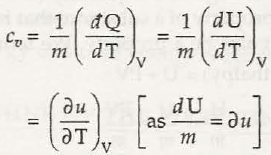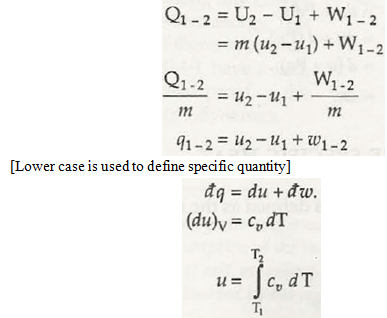In case of an ideal gas which is found at constant pressure would surely take up more heat in order to gain same level of temperature change and that too at constant volume. When there is constant volume heats get added up and finally help to increase temperature. At constant pressure there is some heat that would perform work.Q = nCPΔT

For ideal gas, it is important to apply First Law of Thermodynamics which would say that the heat is equal to:Q = ΔEint + W

When there is constant pressure then, W = P∆V = nR∆T

But, in case of monatomic gas, it is said ΔEint = (3/2)nRΔT, we get:

Q = (3/2)nRΔT + nRΔT = (5/2)nRΔT

So, for a monatomic ideal gas:

CP = (5/2)R

For diatomic and polyatomic ideal gases we get:

Di-atomic: CP = (7/2)R

Polyatomic: CP = 4R

Links of Next Mechanical Engineering Topics:-### Customer Reviews

My Homework Help
Rated 5.0 out of 5 based on 510 customer reviews at